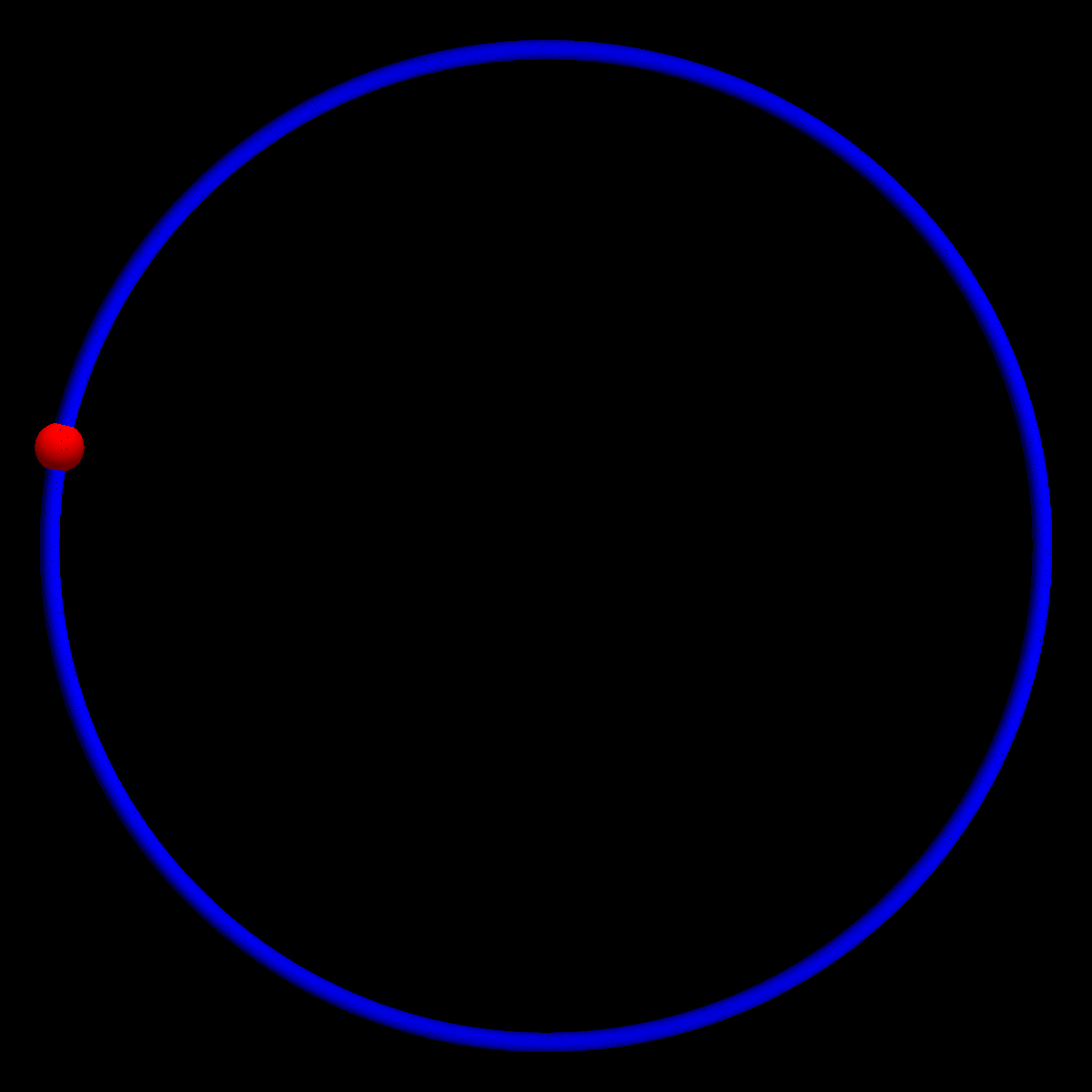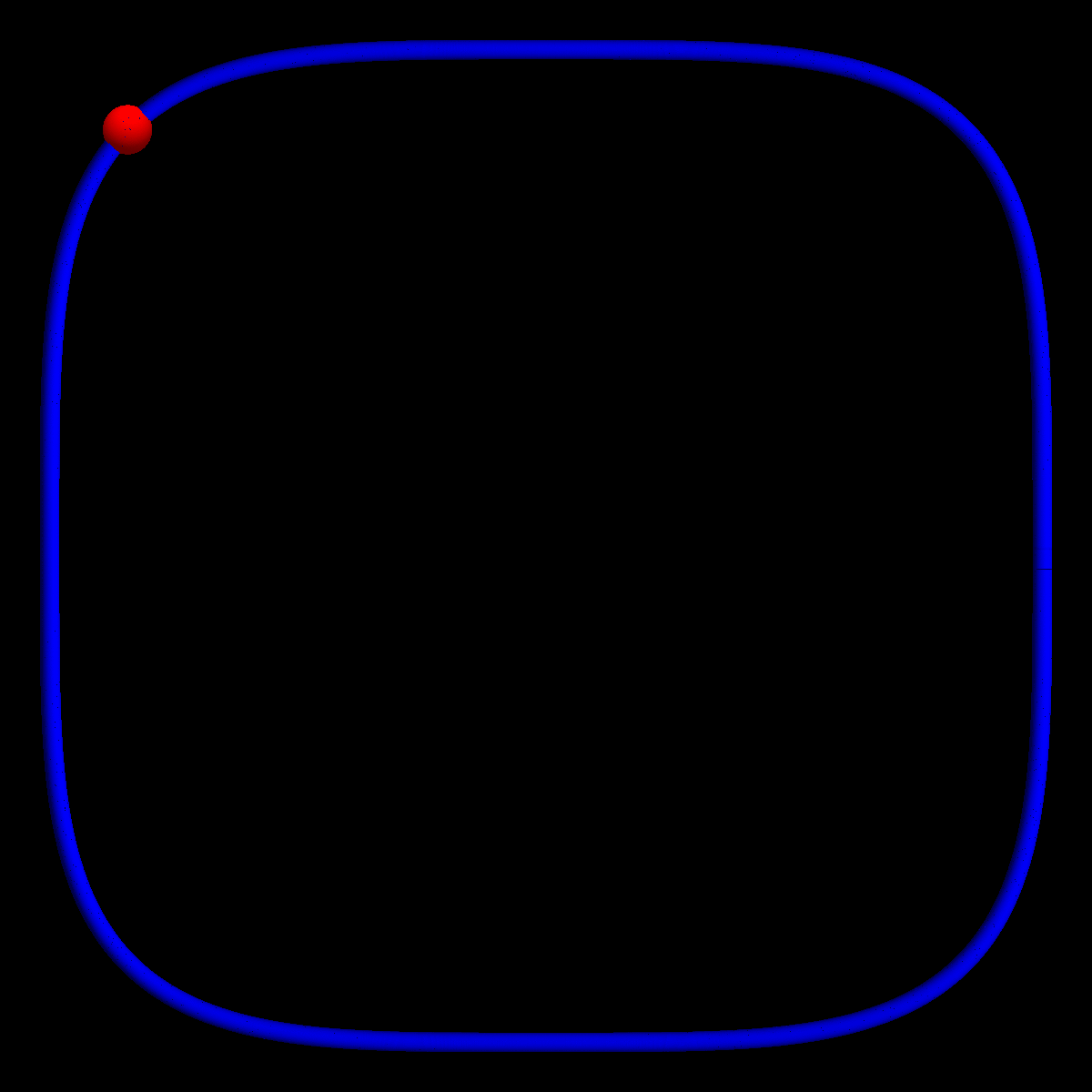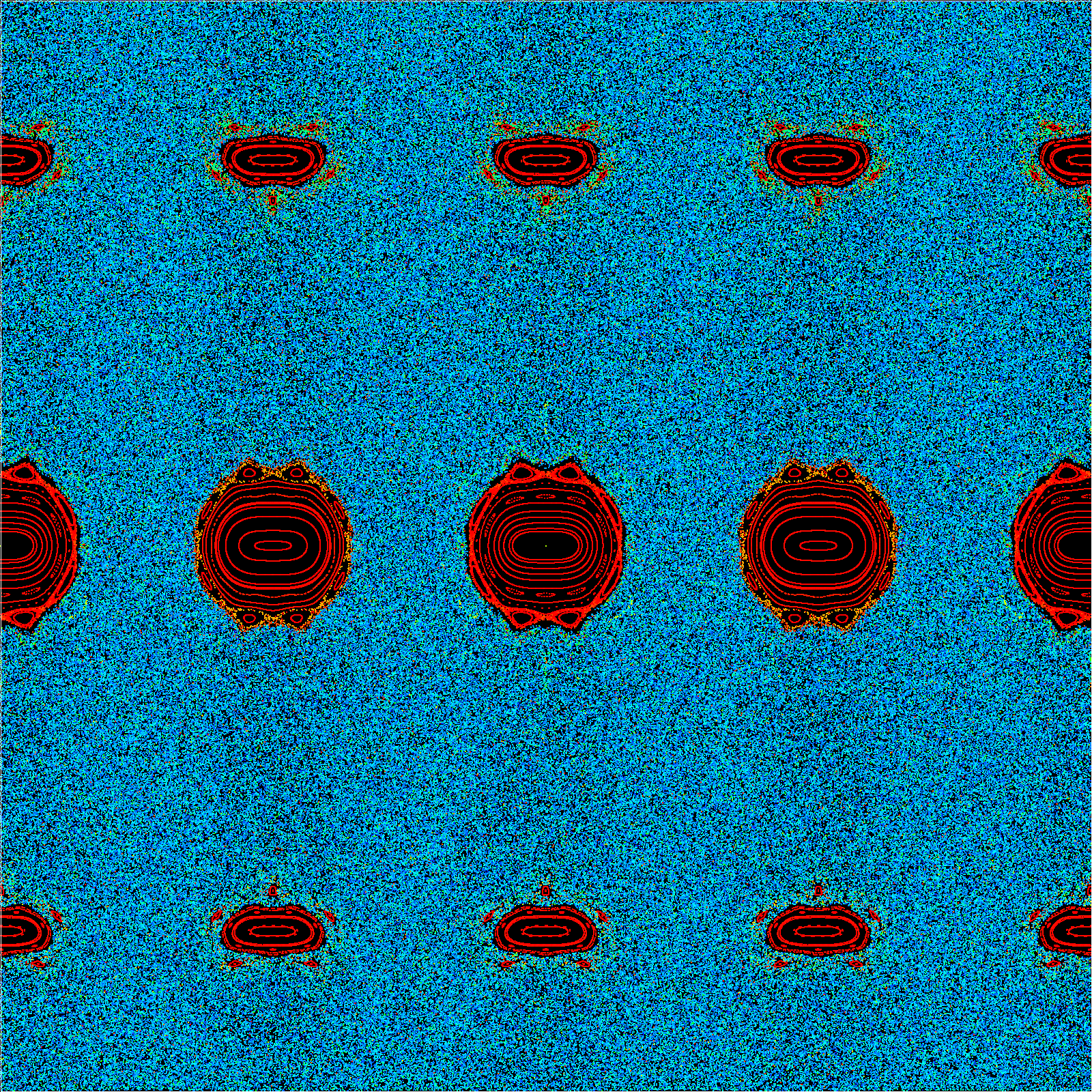# lp Billiard

Here are some orbits of billiards. This billiard has appeared in the Final Exam (PDF). The first one is the circular billiard, p=2, which is an integrable situation. The second one is the case p=4, where we suspect the Kolmogorov Sinai entropy to be positive. Nobody knows how to prove this. In 1995, in a SURF undergraduate research project, Monwhea Jeng worked with me on the problem. We proved here is the article [PDF] the following result

 Theorem (Jeng-Knill): except for p=1, p=infinity or p=2, the topological entropy of the lp billiard is positive.

This result sits on theorems of John Mather (who proved a theorem on billiards with flat points = zero curvature), Andrea Hubacher (who proved a theorem on billiards with unbounded curvature) and Sigurd Angenent who proved that the non-existence of some invariant curves implies positive topological entropy. We also computed the entropy numerically.Here is a newly made phase space picture showing a few orbits. The x-coordinate is the place on the table (here the angular polar coordinate), the y-coordinate shows the cos of the impact angle. We see an obvious dihedral symmetry, which is the 4 fold symmetry of the table as well as the time reversal symmetry, where the orbits are run backwards. The big islands in the middle are the shortest 2-periodic connections which are physical paths on the x-axes or y axes. We see a large blue ``chaotic sea" as well as ``elliptic islands of stability". The reason for the frustratingly hard problem to compute the ``Kolmogorov-Sinai entropy" (mathematically of course, not just measuring as done in the above mentioned paper. Measuring is what physisists do. Mathematicians prove things!) is the presence of these islands. These islands are actually dense in the phase space. The key problem is the non-commutativity of the matrix product, when computing the Jacobean dTn of the n-th iterate, which is a 2x2 matrix. The multiplicative ergodic theorem of Oseledec deals with a non-commutative situation so that we can not just use an ergodic theorem to compute the exponential growth rate of ||dTn|| as in one dimensions where one can write this as ||dT(Tn-1x)|| .... ||dT(x)||. When I was young, I threw essentially every mathematics I could find at this problem ("Anosov-Woitokowski cone methods", "transport approaches", "subharmonicity", "random Schroedinger operators" (Thouless), "isospectral Toda deformations" (of the associated Jacobi operators), "multi-linear algebra", "determinants" or "cocycle deformations", everything failed). It appears to need a new idea. It might even be that the entropy is zero and that the measurements do fool us (which I personally do not believe to be the case). I think the l4 table has positive Kolmogorov-Sinai entropy.